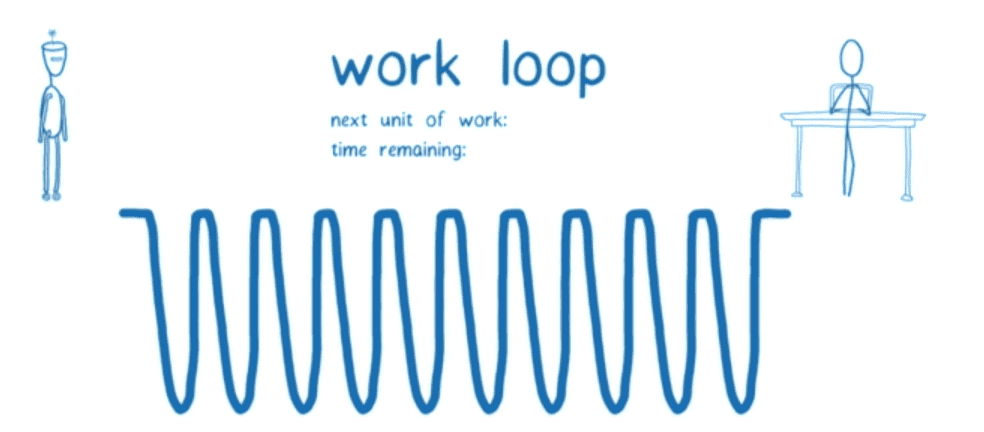## 基础

Fiber的架构有两个主要阶段：协调/渲染和提交。在源码中，协调阶段通常被称为“渲染阶段”。这是React遍历组件树的阶段，并且：

• 更新状态和属性
• 调用生命周期钩子
• 获取组件的`children`
• 将它们与之前的`children`进行对比
• 并计算出需要执行的DOM更新

`Class Component` React需要实例化一个类，然而对于`Functional Component`却不需要。如果有兴趣，在这里

``````requestIdleCallback((deadline)=>{
});
``````

`requestIdleCallback` 实际上有点过于严格，并且执行频次不足以实现流畅的UI渲染，因此React团队必须实现自己的版本

``````requestIdleCallback((deadline) => {
// 当我们有时间时，为组件树的一部分执行工作
nextComponent = performWork(nextComponent);
}
});
``````

## 为什么堆栈与React相关？

`render`函数表示为对象。你可以把它们想象成组件实例：

``````const a1 = {name: 'a1'};
const b1 = {name: 'b1'};
const b2 = {name: 'b2'};
const b3 = {name: 'b3'};
const c1 = {name: 'c1'};
const c2 = {name: 'c2'};
const d1 = {name: 'd1'};
const d2 = {name: 'd2'};

a1.render = () => [b1, b2, b3];
b1.render = () => [];
b2.render = () => [c1];
b3.render = () => [c2];
c1.render = () => [d1, d2];
c2.render = () => [];
d1.render = () => [];
d2.render = () => [];
``````

React需要迭代树并为每个组件执行工作。为了简化，要做的工作是打印当前组件的名字和获取它的children。下面是我们如何通过递归来完成它。

## 递归遍历

``````walk(a1);

function walk(instance) {
doWork(instance);
const children = instance.render();
children.forEach(walk);
}

function doWork(o) {
console.log(o.name);
}
``````

`a1, b1, b2, c1, d1, d2, b3, c2`

## 链表遍历

• child — 第一个子节点的引用
• sibling — 第一个兄弟节点的引用
• return — 父节点的引用

``````class Node {
constructor(instance) {
this.instance = instance;
this.child = null;
this.sibling = null;
this.return = null;
}
}
``````

``````function link(parent, elements) {
if (elements === null) elements = [];

parent.child = elements.reduceRight((previous, current) => {
const node = new Node(current);
node.return = parent;
node.sibling = previous;
return node;
}, null);

return parent.child;
}
``````

``````const children = [{name: 'b1'}, {name: 'b2'}];
const parent = new Node({name: 'a1'});

// 下面两行代码的执行结果为true
console.log(child.instance.name === 'b1');
console.log(child.sibling.instance === children);
``````

``````function doWork(node) {
console.log(node.instance.name);
const children = node.instance.render();
}
``````

``````function walk(o) {
let root = o;
let current = o;

while (true) {
// 为节点执行工作，获取并连接它的children
let child = doWork(current);

// 如果child不为空, 将它设置为当前活跃节点
if (child) {
current = child;
continue;
}

// 如果我们回到了根节点，退出函数
if (current === root) {
return;
}

// 遍历直到我们发现兄弟节点
while (!current.sibling) {

// 如果我们回到了根节点，退出函数
if (!current.return || current.return === root) {
return;
}

// 设置父节点为当前活跃节点
current = current.return;
}

// 如果发现兄弟节点，设置兄弟节点为当前活跃节点
current = current.sibling;
}
}
``````

Fiber是堆栈的重新实现，专门用于React组件。你可以将单个Fiber视为一个虚拟堆栈帧。

``````function walk(o) {
let root = o;
let current = o;

while (true) {
...

current = child;
...

current = current.return;
...

current = current.sibling;
}
}
``````

## React中的工作循环

``````function workLoop(isYieldy) {
if (!isYieldy) {
// Flush work without yielding
while (nextUnitOfWork !== null) {
nextUnitOfWork = performUnitOfWork(nextUnitOfWork);
}
} else {
// Flush asynchronous work until the deadline runs out of time.
while (nextUnitOfWork !== null && !shouldYield()) {
nextUnitOfWork = performUnitOfWork(nextUnitOfWork);
}
}
}
``````